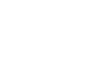How can I calculate my lucky number using my DOB? - letsdiskussVikas joshi

Sales Executive in ICICI Bank | Posted | Astrology

# How can I calculate my lucky number using my DOB?

0
0

Content Writer | Posted

A number which brings good luck and prosperity for you is called Lucky Number in Numerology. There a lot of ways to calculate your lucky number, but the most common and famous is based on your date of birth.

One can determine his/her Lucky number using their date of birth. For an example if your date of birth is 17-12-1986.

1. Reduce the month, day, and year to single digits.

2. It is okay for some numbers to take multiple reductions. In such cases, repeat the same technique until a single digit or Master Number is achieved.

3. Month: 12 = 1+2 = 3

4.
Day: 17 = 1+7 = 8

5.
Year: 1986 = 1+9+8+6 = 24 = 2+4 = 6

6. Now, add the all three results together to make it to a single digit number: 3+8+6 = 17 = 1+ 7 = 8.

Therefore, in case of December 17, 1986, we get lucky number is 8.1
0